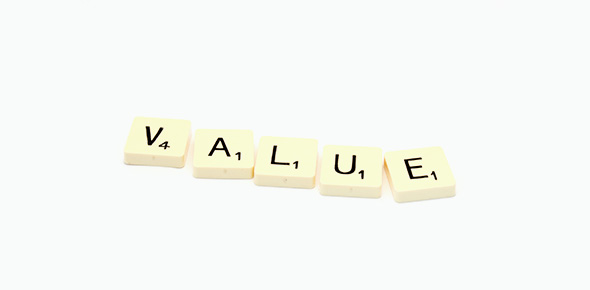# Place Value Pre-assessment

10 Questions | Total Attempts: 76SettingsIn mathematics, the term “place value” refers to the value of a particular digit in a number, with each place having a value of 10 times the place to its right. What do you know of the concept?

Related Topics
• 1.
In 3,872, which digit is in the ones place?
• 2.
What number has a 1 in the millions place, a 5 in the hundreds place, and a 9 in the ones place?
• 3.
In 4,097, how many hundreds are there?
• 4.
In the number 583, what place is the 8 in?
• 5.
Write the missing number 7,000+400+90+2=
• 6.
Which two is worth 20,000?
• A.

82,690

• B.

24,287

• C.

14,428

• D.

2

• 7.
What is 3,000+500+20 in standard form?
• A.

5,320

• B.

3,520

• C.

2,530

• D.

352

• 8.
What number has 9 thousands, no hundreds, and 9 ones?
• A.

9,009

• B.

9,090

• C.

9,900

• D.

9,000

• 9.
What is the value of the underlined digit 4,689,012
• A.

Millions

• B.

Hundred Thousands

• C.

Hundreds

• D.

Thousands

• 10.
What digit is in the millions place? 3,478,092
• A.

4

• B.

3

• C.

9

• D.

8